# 模型部署入门教程（三）：PyTorch 转 ONNX 详解

OpenMMLab：模型部署系列教程（一）：模型部署简介

OpenMMLab：模型部署系列教程（二）：解决模型部署中的难题

## `torch.onnx.export` 细解

### 计算图导出方法

TorchScript 是一种序列化和优化 PyTorch 模型的格式，在优化过程中，一个`torch.nn.Module`模型会被转换成 TorchScript 的 `torch.jit.ScriptModule`模型。现在， TorchScript 也被常当成一种中间表示使用。我们在其他文章中对 TorchScript 有详细的介绍（TorchScript 解读（一）：初识 TorchScript – 知乎），这里介绍 TorchScript 仅用于说明 PyTorch 模型转 ONNX的原理。
`torch.onnx.export`中需要的模型实际上是一个`torch.jit.ScriptModule`。而要把普通 PyTorch 模型转一个这样的 TorchScript 模型，有跟踪（trace）和记录（script）两种导出计算图的方法。如果给`torch.onnx.export`传入了一个普通 PyTorch 模型（`torch.nn.Module`)，那么这个模型会默认使用跟踪的方法导出。这一过程如下图所示：``````import torch

class Model(torch.nn.Module):
def __init__(self, n):
super().__init__()
self.n = n
self.conv = torch.nn.Conv2d(3, 3, 3)

def forward(self, x):
for i in range(self.n):
x = self.conv(x)
return x

models = [Model(2), Model(3)]
model_names = ['model_2', 'model_3']

for model, model_name in zip(models, model_names):
dummy_input = torch.rand(1, 3, 10, 10)
dummy_output = model(dummy_input)
model_trace = torch.jit.trace(model, dummy_input)
model_script = torch.jit.script(model)

# 跟踪法与直接 torch.onnx.export(model, ...)等价
torch.onnx.export(model_trace, dummy_input, f'{model_name}_trace.onnx', example_outputs=dummy_output)
# 记录法必须先调用 torch.jit.sciprt
torch.onnx.export(model_script, dummy_input, f'{model_name}_script.onnx', example_outputs=dummy_output) ``````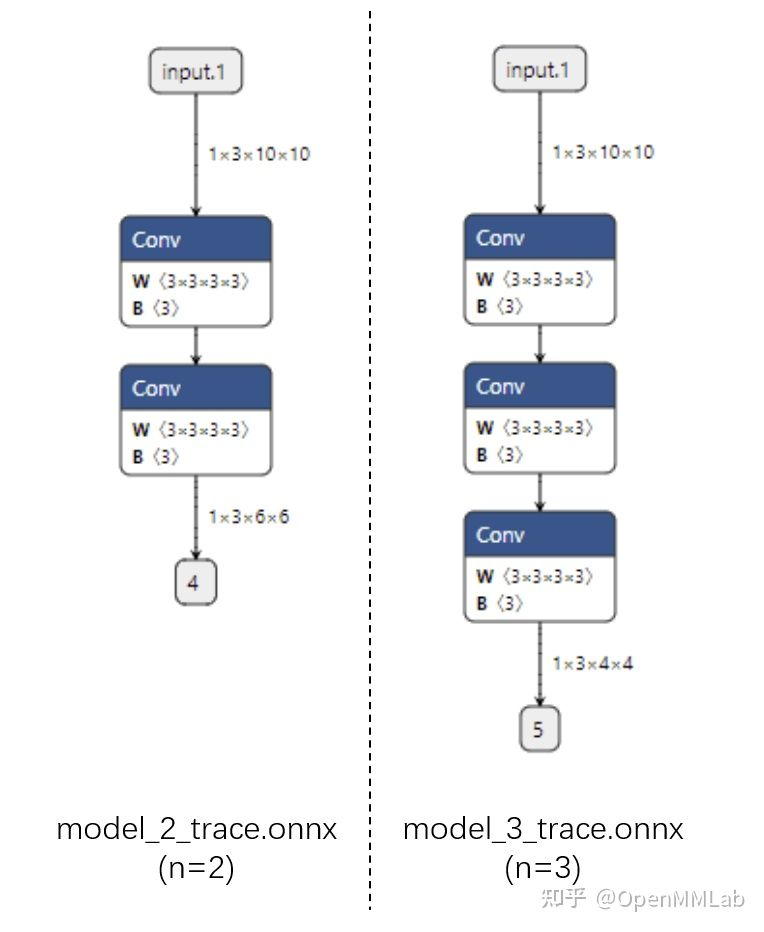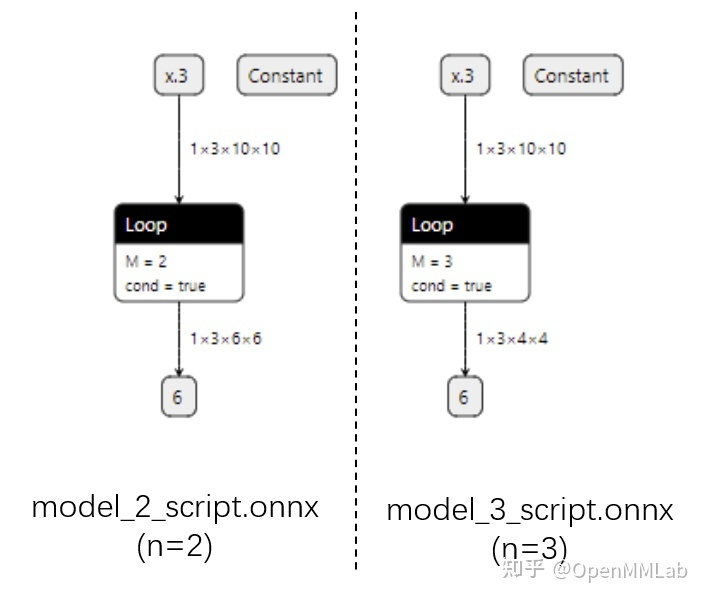### 参数讲解

`torch.onnx.export` 在 `torch.onnx.__init__.py`文件中的定义如下：

``````def export(model, args, f, export_params=True, verbose=False, training=TrainingMode.EVAL,
input_names=None, output_names=None, aten=False, export_raw_ir=False,
operator_export_type=None, opset_version=None, _retain_param_name=True,
do_constant_folding=True, example_outputs=None, strip_doc_string=True,
dynamic_axes=None, keep_initializers_as_inputs=None, custom_opsets=None,
enable_onnx_checker=True, use_external_data_format=False): ``````

export_params

input_names, output_names

ONNX 模型的每个输入和输出张量都有一个名字。很多推理引擎在运行 ONNX 文件时，都需要以“名称-张量值”的数据对来输入数据，并根据输出张量的名称来获取输出数据。在进行跟张量有关的设置（比如添加动态维度）时，也需要知道张量的名字。

opset_version

dynamic_axes

``````import torch

class Model(torch.nn.Module):
def __init__(self):
super().__init__()
self.conv = torch.nn.Conv2d(3, 3, 3)

def forward(self, x):
x = self.conv(x)
return x

model = Model()
dummy_input = torch.rand(1, 3, 10, 10)
model_names = ['model_static.onnx',
'model_dynamic_0.onnx',
'model_dynamic_23.onnx']

dynamic_axes_0 = {
'in' : ,
'out' : 
}
dynamic_axes_23 = {
'in' : [2, 3],
'out' : [2, 3]
}

torch.onnx.export(model, dummy_input, model_names,
input_names=['in'], output_names=['out'])
torch.onnx.export(model, dummy_input, model_names,
input_names=['in'], output_names=['out'], dynamic_axes=dynamic_axes_0)
torch.onnx.export(model, dummy_input, model_names,
input_names=['in'], output_names=['out'], dynamic_axes=dynamic_axes_23) ``````

``````dynamic_axes_0 = {
'in' : ,
'out' : 
} ``````

``````dynamic_axes_0 = {
'in' : {0: 'batch'},
'out' : {0: 'batch'}
} ``````

``````import onnxruntime
import numpy as np

origin_tensor = np.random.rand(1, 3, 10, 10).astype(np.float32)
mult_batch_tensor = np.random.rand(2, 3, 10, 10).astype(np.float32)
big_tensor = np.random.rand(1, 3, 20, 20).astype(np.float32)

inputs = [origin_tensor, mult_batch_tensor, big_tensor]
exceptions = dict()

for model_name in model_names:
for i, input in enumerate(inputs):
try:
ort_session = onnxruntime.InferenceSession(model_name)
ort_inputs = {'in': input}
ort_session.run(['out'], ort_inputs)
except Exception as e:
exceptions[(i, model_name)] = e
print(f'Input[{i}] on model {model_name} error.')
else:
print(f'Input[{i}] on model {model_name} succeed.') ``````

``````Input on model model_static.onnx succeed.
Input on model model_static.onnx error.
Input on model model_static.onnx error.
Input on model model_dynamic_0.onnx succeed.
Input on model model_dynamic_0.onnx succeed.
Input on model model_dynamic_0.onnx error.
Input on model model_dynamic_23.onnx succeed.
Input on model model_dynamic_23.onnx error.
Input on model model_dynamic_23.onnx succeed. ``````

``````print(exceptions[(1, 'model_static.onnx')])

# output
# [ONNXRuntimeError] : 2 : INVALID_ARGUMENT : Got invalid dimensions for input: in for the following indices index: 0 Got: 2 Expected: 1 Please fix either the inputs or the model. ``````

### 使用提示

``````import torch

class Model(torch.nn.Module):
def __init__(self):
super().__init__()
self.conv = torch.nn.Conv2d(3, 3, 3)

def forward(self, x):
x = self.conv(x)
if torch.onnx.is_in_onnx_export():
x = torch.clip(x, 0, 1)
return x ``````

PyTorch 转 ONNX 的跟踪导出法是不是万能的。如果我们在模型中做了一些很“出格”的操作，跟踪法会把某些取决于输入的中间结果变成常量，从而使导出的 ONNX 模型和原来的模型有出入。以下是一个会造成这种“跟踪中断”的例子：

``````class Model(torch.nn.Module):
def __init__(self):
super().__init__()

def forward(self, x):
x = x * x.item()
return x, torch.Tensor([i for i in x])

model = Model()
dummy_input = torch.rand(10)
torch.onnx.export(model, dummy_input, 'a.onnx') ``````

## PyTorch 对 ONNX 的算子支持

• 该算子可以一对一地翻译成一个 ONNX 算子。
• 该算子在 ONNX 中没有直接对应的算子，会翻译成一至多个 ONNX 算子。
• 该算子没有定义翻译成 ONNX 的规则，报错。
• 那么，该如何查看 PyTorch 算子与 ONNX 算子的对应情况呢？由于 PyTorch 算子是向 ONNX 对齐的，这里我们先看一下 ONNX 算子的定义情况，再看一下 PyTorch 定义的算子映射关系。

### ONNX 算子文档

ONNX 算子的定义情况，都可以在官方的算子文档中查看。这份文档十分重要，我们碰到任何和 ONNX 算子有关的问题都得来”请教“这份文档。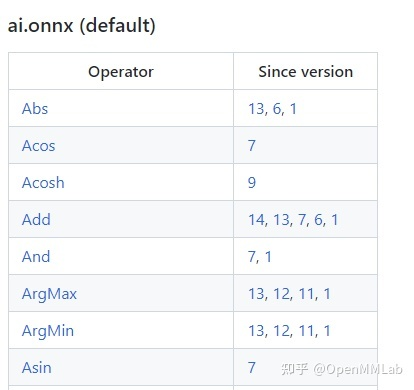这份文档中最重要的开头的这个算子变更表格。表格的第一列是算子名，第二列是该算子发生变动的算子集版本号，也就是我们之前在`torch.onnx.export`中提到的`opset_version`表示的算子集版本号。通过查看算子第一次发生变动的版本号，我们可以知道某个算子是从哪个版本开始支持的；通过查看某算子小于等于`opset_version`的第一个改动记录，我们可以知道当前算子集版本中该算子的定义规则。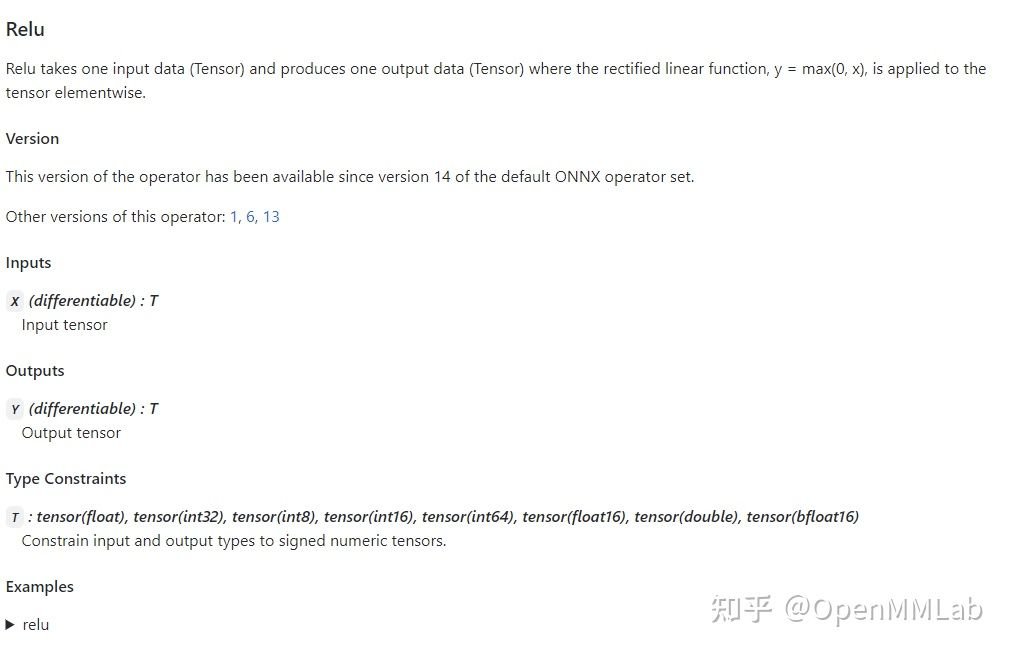通过点击表格中的链接，我们可以查看某个算子的输入、输出参数规定及使用示例。比如上图是 Relu 在 ONNX 中的定义规则，这份定义表明 Relu 应该有一个输入和一个输入，输入输出的类型相同，均为 tensor。

### PyTorch 对 ONNX 算子的映射

在 PyTorch 中，和 ONNX 有关的定义全部放在 `torch.onnx`目录中，如下图所示：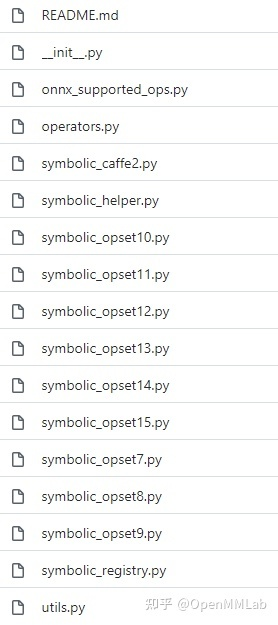其中，`symbolic_opset{n}.py`（符号表文件）即表示 PyTorch 在支持第 n 版 ONNX 算子集时新加入的内容。我们之前讲过， bicubic 插值是在第 11 个版本开始支持的。我们以它为例来看看如何查找算子的映射情况。
首先，使用搜索功能，在`torch/onnx`文件夹搜索"bicubic"，可以发现这个这个插值在第 11 个版本的定义文件中：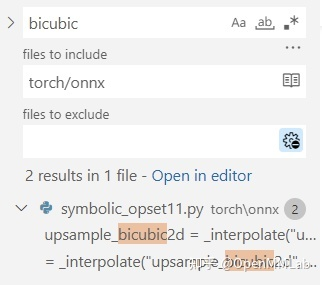之后，我们按照代码的调用逻辑，逐步跳转直到最底层的 ONNX 映射函数：

``````upsample_bicubic2d = _interpolate("upsample_bicubic2d", 4, "cubic")

->

def _interpolate(name, dim, interpolate_mode):
return sym_help._interpolate_helper(name, dim, interpolate_mode)

->

def _interpolate_helper(name, dim, interpolate_mode):
def symbolic_fn(g, input, output_size, *args):
...

return symbolic_fn ``````

最后，在`symbolic_fn`中，我们可以看到插值算子是怎么样被映射成多个 ONNX 算子的。其中，每一个`g.op`就是一个 ONNX 的定义。比如其中的 `Resize` 算子就是这样写的：

``````return g.op("Resize",
input,
empty_roi,
empty_scales,
output_size,
coordinate_transformation_mode_s=coordinate_transformation_mode,
cubic_coeff_a_f=-0.75,  # only valid when mode="cubic"
mode_s=interpolate_mode,  # nearest, linear, or cubic
nearest_mode_s="floor")  # only valid when mode="nearest" ``````

通过在前面提到的ONNX 算子文档中查找 Resize 算子的定义，我们就可以知道这每一个参数的含义了。用类似的方法，我们可以去查询其他 ONNX 算子的参数含义，进而知道 PyTorch 中的参数是怎样一步一步传入到每个 ONNX 算子中的。
掌握了如何查询 PyTorch 映射到 ONNX 的关系后，我们在实际应用时就可以在 `torch.onnx.export()``opset_version`中先预设一个版本号，碰到了问题就去对应的 PyTorch 符号表文件里去查。如果某算子确实不存在，或者算子的映射关系不满足我们的要求，我们就可能得用其他的算子绕过去，或者自定义算子了。

## 总结

在这篇教程中，我们系统地介绍了 PyTorch 转 ONNX 的原理。我们先是着重讲解了使用最频繁的 torch.onnx.export函数，又给出了查询 PyTorch 对 ONNX 算子支持情况的方法。通过本文，我们希望大家能够成功转换出大部分不需要添加新算子的 ONNX 模型，并在碰到算子问题时能够有效定位问题原因。具体而言，大家读完本文后应该了解以下的知识：

• 跟踪法和记录法在导出带控制语句的计算图时有什么区别。
• `torch.onnx.export()`中该如何设置 `input_names, output_names, dynamic_axes`
• 使用 `torch.onnx.is_in_onnx_export()`来使模型在转换到 ONNX 时有不同的行为。
• 如何查询 ONNX 算子文档（https://github.com/onnx/onnx/blob/main/docs/Operators.md）。
• 如何查询 PyTorch 对某个 ONNX 版本的新特性支持情况。
• 如何判断 PyTorch 对某个 ONNX 算子是否支持，支持的方法是怎样的。
• 这期介绍的知识比较抽象，大家会不会觉得有点“水”？没关系，下一期教程中，我们将以给出代码实例的形式，介绍多种为 PyTorch 转 ONNX 添加算子支持的方法，为大家在 PyTorch 转 ONNX 这条路上扫除更多的障碍。敬请期待哦！

## 练习练习

1. Asinh 算子出现于第 9 个 ONNX 算子集。PyTorch 在 9 号版本的符号表文件中是怎样支持这个算子的？
2. BitShift 算子出现于第11个 ONNX 算子集。PyTorch 在 11 号版本的符号表文件中是怎样支持这个算子的？
3. 第一篇教程中，我们讲过 PyTorch （截至第 11 号算子集）不支持在插值中设置动态的放缩系数。这个系数对应 `torch.onnx.symbolic_helper._interpolate_helper``symbolic_fn`的 Resize 算子映射关系中的哪个参数？我们是如何修改这一参数的？

练习的答案会在下期教程中揭晓~快一起动手试试吧~

欢迎大家在 MMDeploy 体验~

https://github.com/open-mmlab/mmdeploy​github.com/open-mmlab/mmdeploy

如果我们的分享给你带来一定的帮助，欢迎点赞收藏关注，比心~来源：OpenMMLab